* You are viewing the archive for the ‘QA: How Light Constant derives other Physical Constants’ Category

## QA: How Light Constant derives other Physical Constants

Up to now, we have been working on Base-9. However, in the posting, QA: Gnomonic-Expansion-Contraction-Spiral, there is a mixture of Base-9 and Base-10 arithmetic. The arithmetic used in generating the Physical Constants in the posting, QA: Physical Constant generated by φ (Phi), is definitely Base-10 and in the Information Vacuum. However, their geometric reference frame is in the Logic Vacuum.

To be correct and specific, Base-9 is used, defined, and created in formulating the Logic-Information potentials within the Logic Vacuum, e.g.,

• the one dimensional symbols (e.g., lines and line diagrams),
• the two dimensional geometry (e.g., the gnomons),
• the numbers 1 to 8 in the Pre-Heaven Octagon.

On the other hand, Base-10 is is used, defined and created in formulating the Information-Energy Potentials in the Information Vacuum, e.g.,

• the Physical Constants
• the Fibonacci Series
• the numbers 1, 2, 3, 4, 6, 7, 8, 9 in the Post-Heaven Octagon. Here 5 is hidden in the middle to induce stability.

[Let me digress briefly to describe the numbers in the Post Heaven Octagon, whose number arrangements are shown below.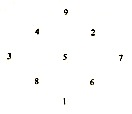Notice, the sum of the diagonals is not 9 as in the pre-heaven octagon, but 15 in base-10 and 6 in base-9. Thus, the post-heaven octagon is directly connected to us humans because the number for human is 6. Yet it is also connected to the Logic-Information crossover onto Information-Energy (or from the Logic Vacuum onto the Information Vacuum). It is this binding action that the physical constants are fine-tuned for intelligent life. See postings, “QA: 6-Days of Creation IIand “QA: What is the Gene” for connections between this binding of pre-post heaven octagons and Creations.]

Thus, the crossover between Base-9 and Base-10 is at the Creation of the Post Heaven Octagon. But remember that we are dealing with the Vacuums, which are non-local and contain no space and no time plus they are interconnected and sometimes entangled depending upon the Observer-Observed interactions with each of their frames of reference.

With this clarification, we can now illustrate the Base-9 / Base-10 interconnections between the pre-heaven and the post-heaven octagons at crossover from the Logic onto the Information Vacuums. Before I begin with the derivation, the following statements are of importance. These statements have to do with the answer to why two derivations of the Physical Constants: one from φ (Phi) with their specific geometrical frames of reference and the other from Base-9 / Base-10 interactive arithmetic with their specific arithmetical frames of reference. The Answer:

• The two derivations suggest that this Universe is formed by the binding between arithmetic and geometry at the Crossover between the Logic Vacuum and the Information Vacuum
• This binding is a necessity for the Collapses of the Information-Energy onto Energy-Mass
• So three-dimensional Space-Energy formation can happen

Although both formulations are separately derived, there seems to be no connections between them. Yet, their binding connection is due to the non-local interconnectedness between geometry and arithmetic.

Let us begin the derivation by expanding the Base-9 pre-heaven octagon numbers. This is shown in the diagram below.

Base-9 <—-> Pre Heaven Octagon Expansion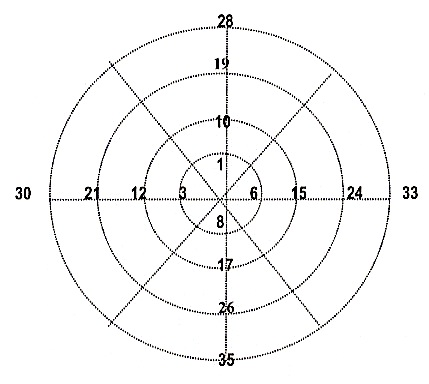Only two diagonals are drawn. Notice that

• 3 + 6 –> 9
• 12 + 15 –> 3 x 9 –>9
• 21 + 24 –> 5 x 9 –>9
• 30 + 33 –> 7 x 9 –> 9

The same calculation can be applied for the other diagonals.

Next, let’s examine a Base-10 concentric circles as shown below.

BASE-10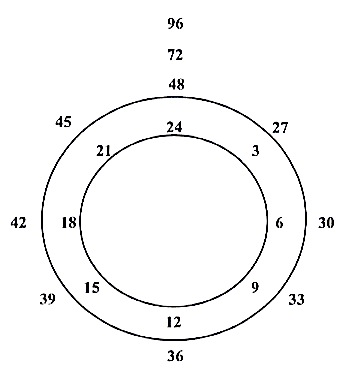Summations of these concentric circles as follow: Counting 25 numbers on each circle, and where the point of intersection between the two circles, which is counted twice as a starting and final numbers. We have the following result for four circles.

• Circle-1: 0 + 2 + … + 24 = 300 = 1 x 300
• Circle-2: 24 + 25 + 26 + … + 48 = 900 = 3 x 300
• Circle-3: 48 + 49 + 50 + … + 75 = 1,500 = 5 x 300
• Circle-4: 72 + 73 + 74 + … + 96 = 2,100 = 7 x 300

Thus, the basic multiplier is 300 multiplying the above sequence of odd numbers of 1, 3, 5, 7… And within this odd number seqence is the prime number set.

If we take the sum of these concentric circles, 10 at a time, we would have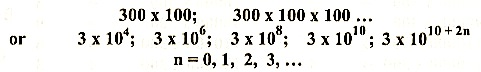Putting the two diagrams together, we have

BASE-9 <—-> BASE-10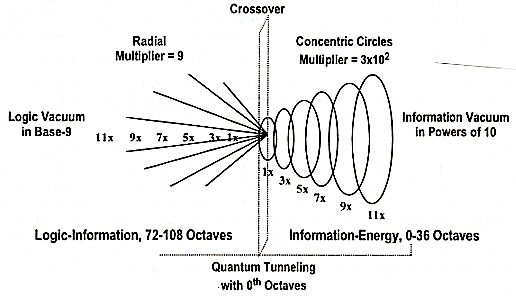By continuing these mappings between Base-9 and Base-10, we obtain the following tables.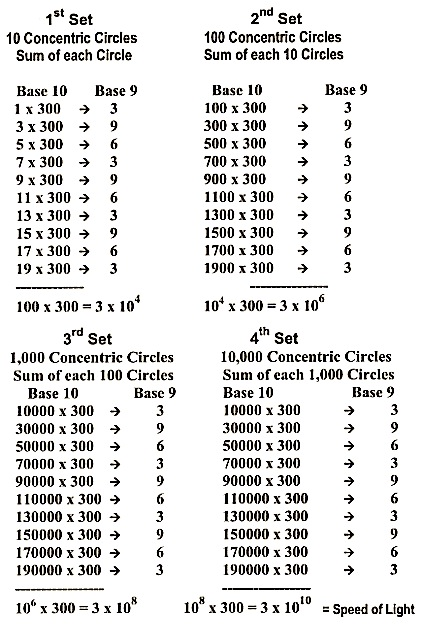On the Base-9 side, starting with the eight with the origin at the center of the Pre-Heaven Octagon, we end up with the Holon 3–9–6. On the Base-10 side, starting with all numbers, we end up with 10 powers of 10, namely 3 x 1010 (the light constant is with respect to the Expansion of Information, not motion).

This concentric expansion also explains the Matter-Being Paradigm’s view on the inflationary part of the universe. With this light constant, we can now derive the other Physical Constants, which is shown below.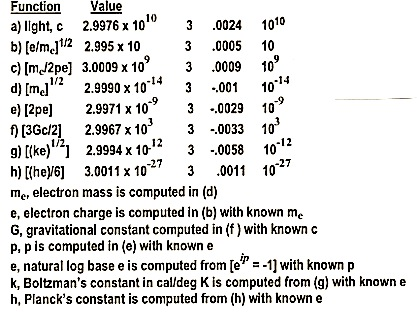The powers of 10 in these constants are the gnomonic expansion-contraction in the numbers of concentric circles. Notice, these concentric circles can also be viewed as generating the gnomonic spirals in the sense of information rather than motion. The plus or minus differences from the number 3 represent the crossover errors.